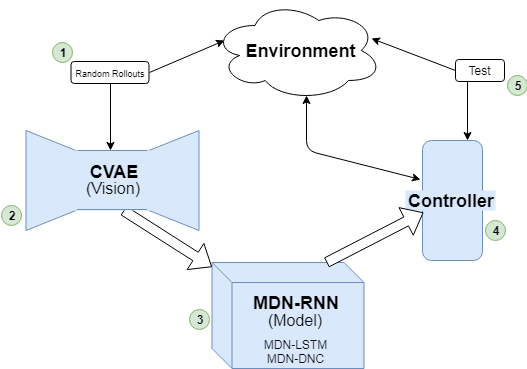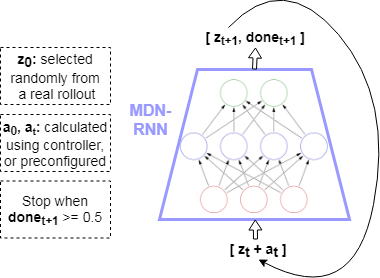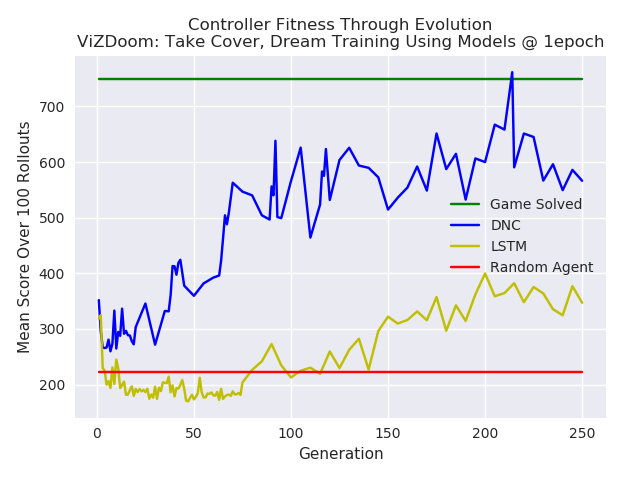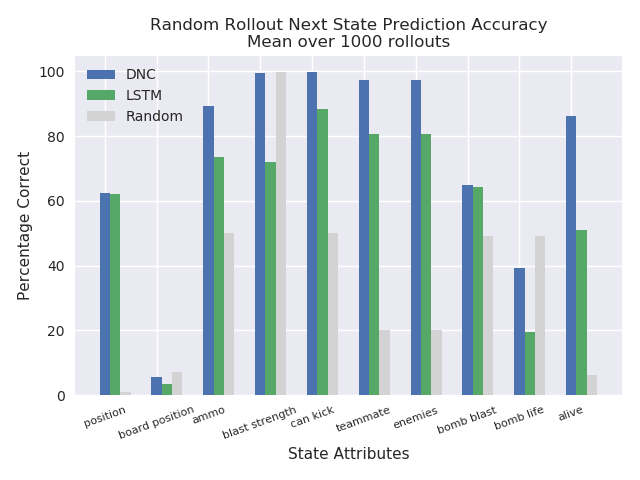## Probabilistic Model-Based Reinforcement Learning Using The Differentiable Neural Computer

My experiments found that a model learned in a Differentiable Neural Computer outperformed a vanilla LSTM based model, on two gaming environments.

➡ Thesis PDF: http://adeel.io/MSc_AI_Thesis.pdf

## Introduction

For my MSc Artificial Intelligence at the University of Edinburgh, my dissertation included 4 months of research. I investigated the use of the Differentiable Neural Computer (DNC) for model-based Reinforcement Learning / Evolution Strategies. A predictive, probabilistic model of the environment was learned in a DNC, and used to train a controller in video gaming environments to maximize rewards (score).

The difference between Reinforcement Learning (RL) and Evolution Strategies (ES) are detailed here. However, in this post and my dissertation, the two are used interchangeably as either is used to accomplish the same goal — given an environment (MDP or partially observable MDP), learn to maximize the cumulative rewards in the environment. The focus is rather on learning a model of the environment, which can be queried while training an ES or RL agent.

The authors of the DNC conducted some simple RL experiments using the DNC, given coded states. However, to the best of my knowledge, this is the first time the DNC was used in learning a model of the environment entirely from pixels, in order to train a complex RL or ES agent. The experiments I conducted showed the DNC outperforming Long Short Term Memory (LSTM) used similarly to learn a model of the environment.

## Learning a Model

The model architecture is borrowed from the World Models framework (see my World Models implementation onGitHub). Given a state in an environment at timestep t,$s_t$, and an action$a_t$ performed at that state, the task of the model is to predict the next state$s_{t+1}$. Thus the input to the model is$[s_t + a_t]$, which produces output (prediction)$s_{t+1}$. Note that the states in this case consist of frames from the game at each timestep consisting of pixels. These states are compressed down to latent variables z using a Convolutional Variational Autoencoder (CVAE), therefore more specifically the model maps$[z_t + a_t] => z_{t+1}$.

The model consists of a Recurrent Neural Network, such as LSTM, that outputs the parameters of a Mixture Density Model — in this cased, a mixture of Gaussians. This type of architecture is known as a Mixture Density Network (MDN), where a neural network is used to output the parameters of a Mixture Density Model. My blog post on MDNs goes into more details. When coupled with a Recurrent Neural Network (RNN), the architecture is known as MDN-RNN.

Thus, in learning a model of an environment, the output of the MDN-RNN “model” is not simply$z_{t+1}$, but the parameters of a Gaussian Mixture Model ($\alpha, \mu, \sigma$) which are then used to sample the prediction of the next state$z_{t+1}$. This allows the model to be more powerful by becoming probabilistic, and encode stochastic environments where the next state after a given state and action can be one of multiple.

For the experiments I conducted, the architecture of the model used a DNC where the RNN is used in World Models, thus, the model is composed of a MDN-DNC. Simply, the recurrent layers used in the MDN are replaced with a DNC (which itself contains recurrent layers that are additionally coupled with external memory).

The hypothesis was that using the DNC instead of vanilla RNNs such as LSTM, will allow for a more robust and algorithmic model of the environment to be learned, thus allowing the agent to perform better. This would particularly be true in complex environments with long term dependencies (meaning, a state perhaps hundreds or thousands of timesteps ago needs to be kept in context for another state down the line).

## Experiments And ResultsExperimentation schematic, based on the World Models framework.

The model of the environment learned is then used to train an RL agent. More specifically, features from the model are used, and in the case of the World Models framework, this consists of the hidden and cell states$h_t, c_t$ of the LSTM layers of the model at every timestep. These “features” of the model, coupled with the compressed latent representation of the environment state, z, at a given timestep t is used as input to a controller. Thus, the controller takes as input$[z_t + h_t + c_t]$ to output action$a_t$ to be taken to achieve a high reward. CMA-ES (more details in my blog post on CMA-ES in Python) was used to train the controller in my experiments.

The games the MDN-DNC was tested on were ViZDoom: Take Cover and Pommerman. For either game, a series of experiments were conducted to compare the results with a model of the environment learned in a MDN-DNC versus a MDN-LSTM.

### ViZDoom: Take Cover

In the case of ViZDoom: Take Cover, a predictive model was trained in the environment using MDN-LSTM and MDN-DNC. Each was trained for 1 epoch, on random rollouts of 10,000 games which recorded the frames from the game (pixels) and actions taken at each timestep. The model was then used to train a CMA-ES controller. Note that the controllers were trained in the “dream” environment simulated by the model, as done in World Models.A simulation of the environment — “dream” — where the controller is used to train using the learned model only, rather than the actual environment.

The controllers were tested in the environment throughout the generations for a 100 rollouts at each test. The results are plotted below. The MDN-DNC based controller clearly outperformed the MDN-LSTM based controller, and solved the game (achieving a mean score of 750 over 100 rollouts).Comparison of a DNC based model versus a LSTM based model, used for training a controller in ViZDoom: Take Cover. The DNC based controller outperforms the LSTM controller.

### Pommerman

In the case of Pommerman, only the model’s predictions were used to test the capacity of the predictive model learned in a MDN-DNC and a MDN-LSTM. A controller was not trained. This was possible given that the states in Pommerman are coded as integers, rather than pixels. Thus, given$[s_t + a_t]$, the predicted state$[s_{t+1}]$ could be compared with the ground truth state from the actual game for equality, and to measure how many components of the state (position, ammo available, etc) were correctly predicted.

Here again, the MDN-DNC model outperformed the MDN-LSTM model, where both were trained exactly the same way for the same number of epochs. The MDN-DNC was more accurately able to predict the individual components of the next state given a current state and an action.The predictive power of a DNC based model versus a LSTM based model in the Pommerman environment. The DNC based model was able to predict future states more accurately.

## Conclusions

Model-based Reinforcement Learning or Evolution Strategies involve using a model of the environment when training a Reinforcement Learning or Evolution Strategies agent. In my case, the World Models approach to learn a predictive, probabilistic model of the environment in an Mixture Density Network was used. The Mixture Density Network consisted of a Differentiable Neural Computer, which output the parameters of a Gaussian mixture model that were used to sample the next state in a game. My experiments found that a model learned in a Differentiable Neural Computer outperformed a vanilla LSTM based model, on two gaming environments.

Future work should include games with long term memory dependencies, whereas with the experiments performed for this work it is hard to justify there being such dependencies in the ViZDoom: Take Cover and Pommerman environments. Other such environments would perhaps magnify the capabilities of the Differentiable Neural Computer. Also, what exactly is going on in the memory of the Differentiable Neural Computer at each timestep? It would be useful to know what it has learned, and perhaps features from the external memory of the Differentiable Neural Computer itself could be used when training a controller. For example, the Differentiable Neural Computer emits read heads,$r_t$, at each timestep, which are selected from the full memory, and used to produce the output (a prediction of the next state). Perhaps the contents of the read heads, or other portions of the external memory, could provide useful information of the environment if exposed directly to the controller along with the hidden state and cell state of the underlying LSTM.

Full details on this work can be found in my MSc thesis at: http://adeel.io/MSc_AI_Thesis.pdf.

## References

 Alex Graves et al., Hybrid computing using a neural network with dynamic external memory, 2016. https://www.nature.com/articles/nature20101
 David Ha, Jürgen Schmidhuber, World Models, 2018. https://arxiv.org/abs/1803.10122
 Nikolaus Hansen, The CMA Evolution Strategy: A Tutorial, 2016. https://arxiv.org/abs/1604.00772
 Christopher M. Bishop, Mixture Density Networks, 1994. https://publications.aston.ac.uk/373/1/NCRG_94_004.pdf